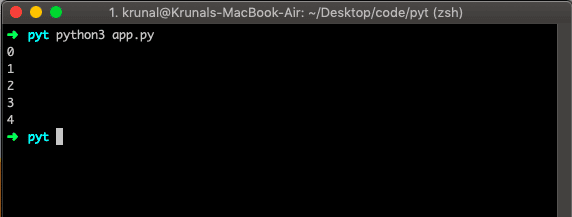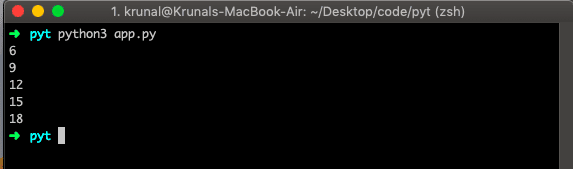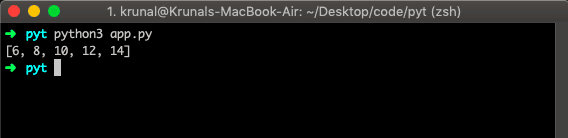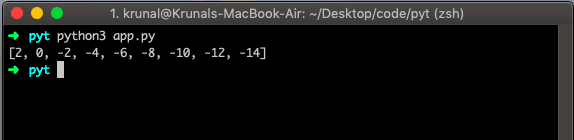# Python range: The Complete Guide

Often you will want to use the range() function when you want to act, say x number of times, where you may or may not care about the index, then this function is useful. For example, sometimes, you may want to iterate over the list or iterable object while having the index available.

## Python range

Python range() is a built-in function that operates several times. The range() function returns an immutable sequence of numbers between a given start integer to a stop integer. In addition, it creates a sequence of numbers from start to stop integer. By the end of this guide, you will understand the basics of range() function in python.

### Syntax

```range(stop)
range(start, stop[, step])```

### Arguments

The range() takes mainly three arguments.

1. start – integer starting from which the sequence of integers is to be returned
2. stop – integer before which the sequence of integers is to be returned.
The range of integers ends at stop – 1.
3. step (Optional) – integer value which determines the increment between each integer in the sequence.

It returns an empty sequence if the stop is negative or 0.

### Example

Let us see the range() method’s example.

```# app.py

app = range(5)
for data in app:
print(data)```

Now, see the output in the console.The range() function returns the sequence of numbers, starting from 0 by default, and increments by 1 (by default), and ends at the specified integer.

Now, let us add a start and stop parameters and see the output.

```# app.py

app = range(6, 11)
for data in app:
print(data)```

So, here we have started from number 6 to 11. But 11 here means 10 because it starts from 0. See the output belLet’s see another example wherehich we add the third parameter step and see the output.

```# app.py

app = range(6, 21, 3)
for data in app:
print(data)```

So, it will print every third integer between 6 to 21.## Create a list of an even number using range()

Let us create a list of an even number using the range() function.

```# app.py

app = range(6, 15, 2)
appList = list(app)
print(appList)```

It will give the following output.## Provide negative step in range() function

Let see the scenario where we provide the negative argument of step and stop in range() method.

```# app.py

app2 = range(2, -15, -2)
appList2 = list(app2)
print(appList2)```In simple terms, the range() function allows you to generate the series of numbers within the given range.

It entirely depends on how many arguments you pass to the function, is it positive or negative arguments or you can decide where that series will begin and end as well as how big the difference will be between one number and the next by defining step parameter.

That’s it for this tutorial.

### 2 thoughts on “Python range: The Complete Guide”

1.Useful example on using range.

2.